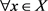﻿ 一类广义非线性Kirchhoff型方程的惯性流形

# 一类广义非线性Kirchhoff型方程的惯性流形Inertial Manifolds for a Class of Nonlinear Generalized Kirchhoff Equation

Abstract: The paper studies the longtime behavior of solutions to the initial boundary value problem (IBVP) for a class of Kirchhoff models: utt+αut-βΔut(Δu‖2)Δu+(1+|u|2)p-1u=f(x). The Inertial manifolds are estimated.

1. 引言

${u}_{tt}+\alpha {u}_{t}-\beta \Delta {u}_{t}-\varphi \left({‖\nabla u‖}^{2}\right)\Delta u+{\left(1+{|u|}^{2}\right)}^{p-1}u=f\left(x\right),\text{ }\Omega ×{R}^{+}$(1.1)

$u\left(x,0\right)={u}_{0}\left(x\right);{u}_{t}\left(x,0\right)={u}_{1}\left(x\right),\text{ }x\in \Omega$(1.2)

${u\left(x,t\right)|}_{\partial \Omega }=0,\text{ }\left(x\right)\in \Omega \text{ }\text{ }$(1.3)

Songmu Zheng和Albert Milani  分别考虑了在一维空间中无粘性的Cahn-Hilliard方程的两种边值问题的奇摄动性。该文证明了基于这两种边值问题的动力系统在相空间 ${H}_{0}^{1}\left(0,\text{π}\right)×{H}^{-1}\left(0,\text{π}\right)$ 存在指数吸引子和惯性流形。

Xu Guigui，Wang Libo和Lin Guoguang  在时滞时间很小的假设下讨论了一类时滞非线性波方程 $\frac{{\partial }^{2}u}{{\partial }^{2}t}+\alpha \frac{\partial u}{\partial t}-\beta \Delta \frac{\partial u}{\partial t}-\Delta u+g\left(u\right)=f\left(x\right)+h\left(t,{u}_{t}\right),\text{\hspace{0.17em}}t>0,\text{\hspace{0.17em}}\alpha >0,\text{\hspace{0.17em}}\beta >0$ 的惯性流形的存在性。

Bin Zhao和Guoguang Lin  考虑了基于Cahn-Hilliard的奇异摄动方程上，具有双重扰动的Cahn-Hilliard方程 $\epsilon \left({u}_{tt}+{u}_{t}\right)-\alpha \Delta {u}_{t}+{\Delta }^{2}{u}_{t}-\Delta {u}^{k}=f,\text{\hspace{0.17em}}k\ge 2$ 的惯性流形的存在性。

Guoguang Lin，Penghui Lv和Ruijin Lou  利用了离散挤压性和谱间隔条件研究了一类广义非线性Kirchhoff-Boussinesq型方程 ${u}_{tt}+\alpha {u}_{t}-\beta \Delta {u}_{t}+{\Delta }^{2}u=div\left(g\left({|\nabla u|}^{2}\right)\nabla u\right)+\Delta h\left(u\right)+f\left(x\right)$ 的指数吸引子和惯性流形。

2. 记号

$\begin{array}{l}{L}^{p}={L}^{p}\left(\Omega \right),\text{ }{W}^{k.p}={W}^{k.p}\left(\Omega \right),\text{ }{H}^{k}={W}^{k,2},\text{ }H={L}^{2},\text{ }‖\cdot ‖={‖\cdot ‖}_{{L}^{2}},\\ {‖\cdot ‖}_{p}={‖\cdot ‖}_{{L}^{p}},\text{ }{V}_{2}={H}^{2}\cap {H}_{0}^{1},\text{ }{V}_{{2}^{\prime }}={V}_{-2},\text{ }{X}_{1}={V}_{2}×{H}_{0}^{1}\end{array}$

${\left(u,v\right)}_{s}=\left({A}^{\frac{s}{4}}u,{A}^{\frac{s}{4}}v\right),\text{ }\text{for}\text{\hspace{0.17em}}{‖u‖}_{{V}_{s}}=‖{A}^{\frac{s}{4}}u‖$

${\lambda }_{1}\left(>0\right)$$-\Delta$$\Omega$ 上带有齐次Dirichlet边界条件的第一特征值。

3. 惯性流形

(H1) $\varphi \in {C}^{1}\left({R}^{+}\right)$${\varphi }^{\prime }\left(s\right)\ge 0$$\varphi \left(0\right)\triangleq {\varphi }_{0}\ge 1$(H2) $f\in H$。则问题(1.1)~(1.3)的解 $\left(u,v\right)$ 满足

${H}_{3}\left(t\right)\le {H}_{3}\left(0\right){\text{e}}^{-\delta t}+\frac{{C}_{5}}{\delta }\left(1-{\text{e}}^{-\delta t}\right)$

$\beta {\int }_{0}^{T}{‖\Delta {u}_{t}‖}^{2}\text{d}s\le {H}_{3}\left(0\right)+{\int }_{0}^{T}{C}_{5}\text{d}s$

1) $\mu$ 是有限维Lipschitz流形，

2) $\mu$ 是正不变的，即 $S\left(t\right)\mu \subset \mu ,t\ge 0$

3) $\mu$ 以指数吸引解轨道，即，存在常数 $\eta >0,C>0$ ，使得

${‖F\left(U\right)-F\left(V\right)‖}_{X}\le {l}_{F}{‖U-V‖}_{X},\text{\hspace{0.17em}}\text{\hspace{0.17em}}U,V\in X$(3.1)

${\Lambda }_{1}=\mathrm{sup}\left\{\mathrm{Re}\lambda |\lambda \in {\sigma }_{1}\right\},\text{\hspace{0.17em}}\text{\hspace{0.17em}}{\Lambda }_{2}=\mathrm{inf}\left\{\mathrm{Re}\lambda |\lambda \in {\sigma }_{2}\right\}$(3.2)

${X}_{i}=span\left\{{w}_{j}|j\in {\sigma }_{i}\right\},\text{\hspace{0.17em}}\text{\hspace{0.17em}}i=1,2$ (3.3)

${\lambda }_{2}-{\lambda }_{1}>4{l}_{F}$(3.4)

${U}_{t}+AU=F\left(U\right),\text{\hspace{0.17em}}\text{\hspace{0.17em}}U\in X$(3.6)

$U=\left(u,v\right)=\left(u,{u}_{t}\right)$$F\left(U\right)=\left(\begin{array}{c}0\\ f-{\left(1+{|u|}^{2}\right)}^{p-1}u\end{array}\right)$ (3.7)$X=\left({H}^{2}\cap {H}_{0}^{1}\right)×{H}_{0}^{1}$

${〈U,V〉}_{X}=\left(\Delta u,\Delta \stackrel{¯}{y}\right)+\left(-\Delta v,\stackrel{¯}{z}\right)$(3.8)

$U=\left(u,v\right),V=\left(y,z\right)$ , $\stackrel{¯}{y},\text{\hspace{0.17em}}\stackrel{¯}{z}$ 分别表示 $y,\text{\hspace{0.17em}}z$ 的共轭。

$U\in D\left(A\right)$ ，计算得

$\begin{array}{c}{〈AU,V〉}_{Z}=-\left(\Delta v,\Delta u\right)+\left(-\Delta \left(-\varphi \Delta u+\alpha v-\beta \Delta v\right),v\right)\\ =-\left(\Delta v,\Delta u\right)+\left(-\varphi \Delta u+\alpha v-\beta \Delta v,-\Delta v\right)\\ =-\left(\varphi -1\right)\left(\Delta v,\Delta u\right)+\alpha {‖\nabla v‖}^{2}+\beta {‖\nabla v‖}^{2}\end{array}$ (3.9)

$AU=\lambda U,\text{\hspace{0.17em}}U=\left(u,v\right)\in X$

$\left\{\begin{array}{l}-v=\lambda u,\\ -\varphi \Delta u+\alpha v-\beta \Delta v=\lambda v,\end{array}$ (3.10)

$\left\{\begin{array}{l}-\varphi \Delta u+\beta \lambda \Delta u=\left(\alpha \lambda -{\lambda }^{2}\right)u,\\ {u|}_{\partial \Omega }=0,\end{array}$ (3.11)

${U}_{k}^{±}=\left({u}_{k},{v}_{k}\right)=\left({u}_{k},-{\lambda }_{k}^{±}{u}_{k}\right)$${u}_{k}\left(x\right)={\left(\frac{2}{\text{π}}\right)}^{\frac{1}{2}}\mathrm{sin}\left(jx\right)$(3.12)

$‖\nabla {u}_{k}‖=j,‖\Delta {u}_{k}‖={j}^{2},‖{u}_{k}‖=1$(3.13)

${\lambda }_{k}^{±}=\frac{\left(\alpha +\beta {j}^{2}\right)±\sqrt{{\left(\alpha +\beta {j}^{2}\right)}^{2}-4\varphi {j}^{2}}}{2}$(3.14)

$2\beta N+1\ge \frac{8l}{\beta -\varphi }$(3.15)

${\sigma }_{1}=\left\{{\lambda }_{j}^{-},{\lambda }_{k}^{±}|\mathrm{max}\left({\lambda }_{j}^{-},{\lambda }_{k}^{±}\right)\le {\lambda }_{N}^{+}\right\}$${\sigma }_{2}=\left\{{\lambda }_{j}^{+}|{\lambda }_{j}^{-}\le {\lambda }_{N}^{+}<{\lambda }_{j}^{+}\right\}$(3.16)

${X}_{1}=span\left\{{v}_{j}^{-},{v}_{k}^{±}|{\lambda }_{j}^{-},{\lambda }_{k}^{±}\in {\sigma }_{1}\right\}$${X}_{2}=span\left\{{v}_{j}^{+}|{\lambda }_{j}^{+}\in {\sigma }_{2}\right\}$(3.17)

${X}_{c}=span\left\{{v}_{j}^{-}|{\lambda }_{j}^{-}\le {\lambda }_{N}^{+}<{\lambda }_{j}^{+}\right\}$

${X}_{k}=span\left\{{v}_{k}^{±}|{\lambda }_{k}^{±}\le {\lambda }_{N}^{+}\right\}$(3.18)

${\lambda }_{j}^{+}{\lambda }_{j}^{-}=\varphi {j}^{2}$

${〈{U}_{j}^{+},{U}_{j}^{-}〉}_{X}={‖\Delta {u}_{j}‖}^{2}+{\lambda }_{j}^{+}{\lambda }_{j}^{-}{‖\nabla {u}_{j}‖}^{2}=\left(1+\varphi \right){j}^{4}$(3.19)

$U\in Z$

$\begin{array}{c}{〈〈U,U〉〉}_{X}=\alpha {‖\nabla u‖}^{2}+\left(\beta -\varphi \right){‖\Delta u‖}^{2}+2\left(v,-\Delta u\right)+{‖\nabla u‖}^{2}\\ \ge \alpha {‖\nabla u‖}^{2}+\left(\beta -\varphi \right){‖\Delta u‖}^{2}-{‖\nabla u‖}^{2}-||\nabla v|{|}^{2}+{‖\nabla u‖}^{2}\\ \ge \left(\alpha -1\right){‖\nabla u‖}^{2}+\left(\beta -\varphi \right){‖\Delta u‖}^{2}\end{array}$

$\alpha \ge 1,\beta >\varphi$ ，所以 ${〈〈U,V〉〉}_{X}\ge 0$

$\begin{array}{c}{〈〈{U}_{j}^{+},{U}_{j}^{-}〉〉}_{X}=\alpha {‖\nabla {u}_{j}‖}^{2}+\left(\beta -\varphi \right){‖\Delta {u}_{j}‖}^{2}-\left({\lambda }_{j}^{+}+{\lambda }_{j}^{-}\right){‖\nabla {u}_{j}‖}^{2}+{\lambda }_{j}^{+}{\lambda }_{j}^{-}{‖\nabla {u}_{j}‖}^{2}\\ =\alpha {j}^{2}+\left(\beta -\varphi \right){j}^{4}-\left(\alpha +\beta {j}^{2}\right){j}^{2}+\varphi {j}^{4}=0\end{array}$

${‖F\left(U\right)-F\left(V\right)‖}_{X}={‖g\left(u\right)-g\left(v\right)‖}_{{H}_{0}^{1}}\le l‖\Delta \left(u-v\right)‖\le \frac{l}{\beta -\varphi }{‖U-V‖}_{X}$

${l}_{F}\le \frac{l}{\beta -\varphi }$

${\lambda }_{N+1}^{+}=\frac{\alpha +\beta {\left(N+1\right)}^{2}+\sqrt{{\left(\alpha +\beta {\left(N+1\right)}^{2}\right)}^{2}-4\varphi {\left(N+1\right)}^{2}}}{2}$

${\lambda }_{N+1}^{+}=\frac{\alpha +\beta {N}^{2}+\sqrt{{\left(\alpha +\beta {N}^{2}\right)}^{2}-4\varphi {N}^{2}}}{2}$$\mu =graph\left(\Phi \right)=\left\{\xi +\varphi \left(\xi \right);\xi \in X\right\}$

NOTES

*通讯作者。

 Temam, R. (1990) Intertial Manifolds. The Mathematical Intelligencer, 12, No. 4.

 Marion, M. (1989) Intertial Manifolds Associated to Partly Dissipative Reaction-Diffusion Systems. Journal of Mathematical Analysis and Applications, 143, 295-326. https://doi.org/10.1016/0022-247X(89)90043-7

 Rezounenko, A.V. (2002) Inertial Manifolds for Retarded Second Order in Time Evolution Equations. Nonlinear Analysis, 51, 1045-1054. https://doi.org/10.1016/S0362-546X(01)00878-1

 Huy, N.T. (2012) Inertial Manifolds for Semi-Linear Parbolic Equations in Admissible Spaces. Journal of Mathematical Analysis and Applications, 386, 894-909. https://doi.org/10.1016/j.jmaa.2011.08.051

 Foias, C. (1988) Inertial Manifolds for Nonlinear Evolutionary Equations. Journal of Differential Equations, 73, 309-353. https://doi.org/10.1016/0022-0396(88)90110-6

 Liu, W.J. and Haller, G. (2004) Inertial Manifolds and Completeness of Eigenmodes for Unsteady Magnetic Dynamos. Physica D, 194, 297-319. https://doi.org/10.1016/j.physd.2003.03.002

 Zheng, S.M. and Milani, A. (2004) Exponential Attractors and Inertial Manifolds for Singular Perturbations of the Cahn-Hilliard Equations. Nonlinear Analysis, 57, 843-877. https://doi.org/10.1016/j.na.2004.03.023

 Xu, G.G., Wang, L.B. and Lin, G.G. (2014) Inertial Manifolds for a Class of the Retarded Nonlinear Wave Equations. Mathematica Applicata, 27, 887-891.

 Zhao, B. and Lin, G.G. (2013) Inertial Manifolds for Dual Perturbations of the Cahn-Hilliard Equations. Far East Journal of Applied Mathematics, 77, 113-136.

 Lin, G.G., Lv, P.H. and Lou, R.J. (2017) Exponential Attractors and Inertial Manifolds for a Class of Nonlinear Generalized Kirchhoff-Boussinesq Model. Far East Journal of Mathematical Sciences, 101, 1913-1945. https://doi.org/10.17654/MS101091913

 Lv, P.H., Lu, J.X. and Lin, G.G. (2016) Global Attractors for a Class of Nonlinear Generalized Kirchhoff Models. Journal of Advances in Mathematics, 12, 6452-6462.

Top